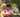### Min Avg Two Slice

January 23, 2020

Min Avg Two Slice

A non-empty array A consisting of N integers is given. A pair of integers (P, Q), such that 0 ≤ P < Q < N, is called a slice of array A (notice that the slice contains at least two elements). The average of a slice (P, Q) is the sum of A[P] + A[P + 1] + ... + A[Q] divided by the length of the slice. To be precise, the average equals (A[P] + A[P + 1] + ... + A[Q]) / (Q − P + 1).

For example, array A such that:
A = 4
A = 2
A = 2
A = 5
A = 1
A = 5
A = 8
contains the following example slices:
• slice (1, 2), whose average is (2 + 2) / 2 = 2;
• slice (3, 4), whose average is (5 + 1) / 2 = 3;
• slice (1, 4), whose average is (2 + 2 + 5 + 1) / 4 = 2.5.
The goal is to find the starting position of a slice whose average is minimal.

Write a function:
function solution(A); that, given a non-empty array A consisting of N integers, returns the starting position of the slice with the minimal average. If there is more than one slice with a minimal average, you should return the smallest starting position of such a slice.

For example, given array A such that:
A = 4
A = 2
A = 2
A = 5
A = 1
A = 5
A = 8
the function should return 1, as explained above.

Write an efficient algorithm for the following assumptions:
• N is an integer within the range [2..100,000];
• each element of array A is an integer within the range [−10,000..10,000].

hint : check slices in two or three elements

``````function solution(A) {
// write your code in JavaScript (Node.js 8.9.4)
let minStart = 0

let sum = A + A // initial start
let minAvg = sum / 2
let avg

for(let i=2; i<A.length; i++){
sum = sum + A[i] // slice of 3
avg = sum / 3
if( minAvg > avg ) {
minAvg = avg
minStart = i-2
}

sum = sum - A[i-2] // slice of 2
avg = sum / 2
if( minAvg > avg ){
minAvg = avg
minStart = i-1
}
}
return minStart
}``````Posted by Tai Lu ( 呂台生 ) who's building useful things.You should follow him on Twitter